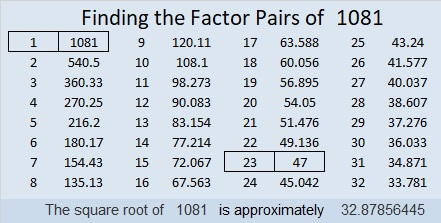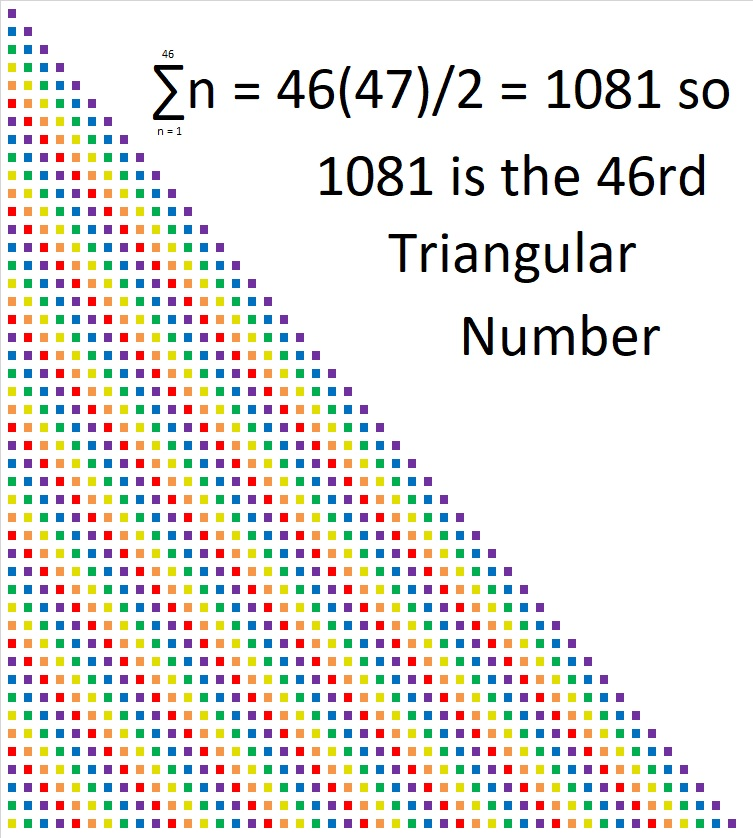# 1081 and Level 1

If you know basic division and multiplication facts for factors 1 to 12, then you can complete this whole puzzle and make it be a multiplication table but with the factors not in their usual places.Print the puzzles or type the solution in this excel file: 12 factors 1080-1086

Here are a few facts about the number 1081:

• 1081 is a composite number.
• Prime factorization: 1081 = 23 × 47
• The exponents in the prime factorization are 1 and 1. Adding one to each and multiplying we get (1 + 1)(1 + 1) = 2 × 2 = 4. Therefore 1081 has exactly 4 factors.
• Factors of 1081: 1, 23, 47, 1081
• Factor pairs: 1081 = 1 × 1081 or 23 × 47
• 1081 has no square factors that allow its square root to be simplified. √1081 ≈ 32.878561081 is the 46th triangular number because 46(47)/2 = 1081
That means that the sum of the numbers from 1 to 46 is 1081:
1 + 2 + 3 + 4 + . . . + 43 + 44 + 45 + 46 = 10811081 is also the sum of eleven consecutive prime numbers:
73 + 79 + 83 + 89 + 97 + 101 + 103 + 107 + 109 + 113 + 127 = 1081

In other bases, 1081 is 3 different palindromes that begin and end with 1:
1L1 in BASE 24 (L is 21 base 10) because 24² + 21(24) + 1 = 1081
1D1 in BASE 27 (D is 13 base 10) because 27² + 13(27) + 1 = 1081
161 in BASE 30 because 30² + 6(30) + 1 = 1081

This site uses Akismet to reduce spam. Learn how your comment data is processed.